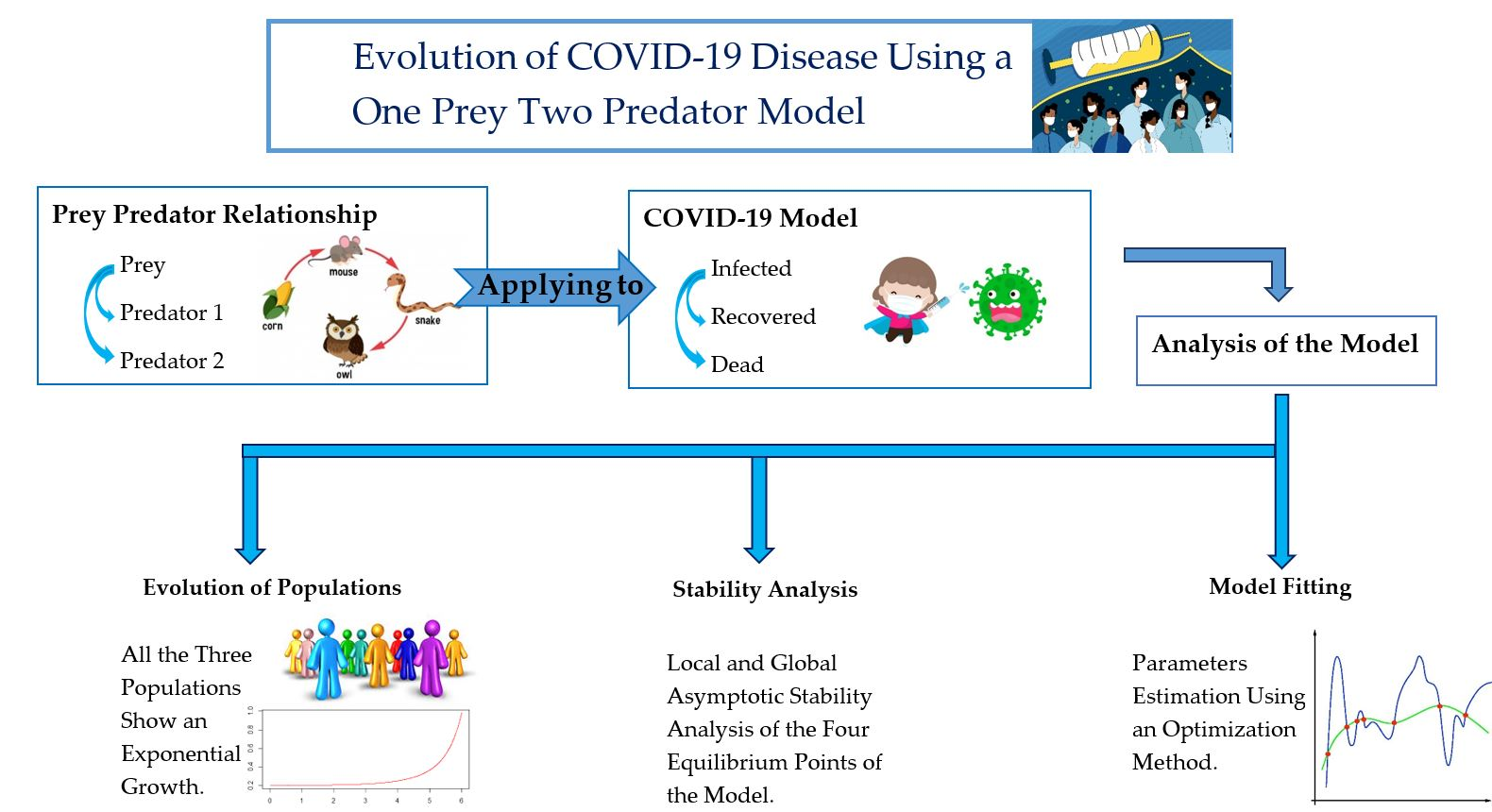# Evolution of COVID-19 Disease Using a One Prey-Two Predator Model

## Authors

• Amila Sudu Ambegedara Department of Mathematics, Faculty of Science, University of Peradeniya
• Asini A. Konpola
• Chathurika S. Gunasekara
• Indika G. Udagedara Department of Mathematics, Faculty of Science, University of Peradeniya

## Abstract

Mathematical modeling is used to understand the dynamics of transmission of infectious diseases such as COVID-19, SARS, Ebola, and Dengue among populations. In this work, a one prey-two predator model has been developed to understand the underlying dynamics of COVID-19 disease transmission. We considered the infected, recovered, and death populations with the fact that an infected person can be transformed into the recovered or death group assuming that the infected ones are the prey, and the other two populations are the two predators in the one prey-two predator model. It was found that the proposed model has four equilibrium points; the vanishing equilibrium point ( ), recovered and death-free equilibrium point ( ), recovered population-free equilibrium point ( ), and the death-free equilibrium point ( ). Stability analysis of the equilibrium points shows that except  all the other equilibrium points are locally asymptotically stable. Global asymptotic stability of the recovered population-free equilibrium point and death-free equilibrium point are also analyzed. Moreover, the existence and uniqueness of the solution were proved. The parameters for the model are estimated from a data set that consists of the total number of infected, recovered, and dead populations worldwide in the year 2020 using the Nelder-Mead optimization method. When the time approaches infinity, the infected population converges to a constant value, the recovered population declines and reaches zero, and the death population attains a constant value. However, some modifications to the system are needed. In future work, measures such as health precautions, vaccinations are needed to be considered for the formulation of the mathematical model.2021-05-31

## How to Cite

Sudu Ambegedara, A., Konpola, A. A., Gunasekara, C. S., & Udagedara, I. G. (2021). Evolution of COVID-19 Disease Using a One Prey-Two Predator Model. Advances in Technology, 1(1), 25–40. https://doi.org/10.31357/ait.v1i1.4888

## Section

Science for Technology